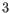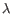# Differential Expression

Two tools are available in the Workbench for calculating differential expressions. The Differential Expression in Two Groups tool performs a statistical differential expression test for a set of Expression Tracks and a set of control tracks. The Differential Expression for RNA-Seq tool performs a statistical differential expression test for a set of Expression Tracks with associated metadata. Both tools use multi-factorial statistics based on a negative binomial Generalized Linear Model (GLM).

 How many replicates do I need? The Differential Expression for RNA-Seq tool is capable of running without replicates, but this is not recommended and the results should be treated with caution. In general it is desirable to have as many biological replicates as possible - typically at least. Replication is important in that it allows the 'within group' variation to be accurately estimated for a gene. In the absence of replication, the Differential Expression for RNA-Seq tool assumes that genes with similar average expression levels have similar variability. Technical or biological replicates? [Auer and Doerge, 2010] illustrates the importance of biological replicates with the example of an alien visiting Earth. The alien wishes to know if men are taller than women. It abducts one man and one woman, and measures their heights several times i.e. performs several technical replicates. However, in the absence of biological replicates, the alien would erroneously conclude that women are taller than men if this was the case in the two abducted individuals.

The use of the GLM formalism allows us to fit curves to expression values without assuming that the error on the values is normally distributed. Similarly to edgeR and DESeq, we assume that the read counts follow a Negative Binomial distribution as explained in [McCarthy et al., 2012]. The Negative Binomial distribution can be understood as a 'Gamma-Poisson' mixture distribution i.e., the distribution resulting from a mixture of Poisson distributions, where the Poisson parameteris itself Gamma-distributed. In an RNA-Seq context, this Gamma distribution is controlled by the dispersion parameter, such that the Negative Binomial distribution reduces to a Poisson distribution when the dispersion is zero.

To learn more about the performance of the Differential Expression Analysis tool in comparison to well-accepted protocols like DEseq, EdgeR, read our benchmark results here: https://www.qiagenbioinformatics.com/blog/discovery/lasting-expressions/.

Subsections